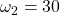# A Simple Model for Realized Volatility

The post has two goals:

(1) Explain how to forecast volatility using a simple Heterogeneous Auto-Regressive (HAR) model. (Corsi, 2002)
(2) Check if higher moments like Skewness and Kurtosis add forecast value to this model.

It will be a high frequency analysis as the data is recorded on minutely basis. The purpose is to construct an accurate proxy for the daily volatility using this data, and forecast ahead using the HAR model.

Heterogeneous Auto-Regressive (HAR) model
The model is created to capture few stylized facts observed in the data. Among others, volatility distribution has fat tails, meaning we observe very high or very low values with relatively high probability. Also, volatility has long memory, so a value ‘long ago’, for example, 20 days ago, may still have an impact on the value we will see tomorrow. We can estimate a very long Auto-regressive model, say 30 lags, but that might lead to over fitting and apart from that, it may not be necessary to allow this kind of flexibility. The model itself is very simple:where,. We can useand, corresponding with one week and one month. That way, we do not need to estimate so many lags.

Win: No need to estimate 30 lags.
lose: Flexibility. We assume a constant influence of all lags in the same “time zone”.

For example, lagged volatility of the order 2 through 7 will have same coefficient. The “…” is there to remind you that you can add more variables that you think can help in prediction. For example, we will add lagged return and the lagged sign of the return, which will add asymmetry effect, like in GJR-Garch or Asymmetric garch models.

In sum, it is a very simple linear regression.

Data for this tutorial can be found here. After saving the data you can load it as follows:

What is loaded is an array named prlev with dimension 390 – 8 – 250 which is 390 – minutes of 8 – (high close, etc.. as in Yahoo.finance) for the 250 – days ending in 22/10/2012. The ticker is SPY.

In order to construct the model we want to create the‘s. I use the intra-day prices and construct what is called the Realiized Range over a time interval of one day. One estimator is the Garman-Klass. Few more can be found here.

So now volat is a vector of 250 observations, each represents the realized volatility for each day.

Next we construct the right hand side which will help to clarify the model above:

This is pretty much all the hard work we have for today. Now it is a matter of simple lm function.

So, we see the effect of volatility clustering, the volatility today, the volatility of past week and past month all have significant impact on the volatility tomorrow. Positive sign means that when they are up, the volatility today, on average, goes up as well.

It might be interesting to see if higher moments help to predict, so let’s incorporate the lagged (intra-day) skewness and kurtosis we collected before.

No, they are not important, that is, once you account for the lagged volatility and allow for asymmetry in the form of lagsign, there is not much added value in the higher moment. At least not prediction value.

References

here is a working paper version of the HAR paper by Corsi (2002).

### 8 comments on “A Simple Model for Realized Volatility”

1.Michael says:

I think you mean the Garman-Klass estimator (http://goo.gl/nTWpM)

1.Eran says:

Of course, thanks.

2.horowo says:

What’s the definition of volatw?

1.Eran says:

Hi, Thanks, I corrected the code.

3.Dan says:

Nice post but I had a couple of issues running the code:
1) The ctc calc “ctc = (prlev[390,4,2:n]-prlev[390,4,1:(n-1)])/prlev[390,4,1:(n-1)]#Create Close to close returns”
looks for n but it is not defined except in the GarmanKlass function.

I set n to
nbrs<-dim(prlev)
n=nbrs
and that seems to work. Is this correct?

2) ret0 in the loop isn't defined – so I set it to ctc – is that correct?
2)volatw is set to NULL then used in the
har0 = lm(volat~volatd+volatw+volatm+lagret0+lagsign ) but it is not part of the loop that sets the the values for the other variables unless I missed something. What should the values be?
Thanks
Dan

1.Eran says:

Hi Dan,

Indeed, “n” is defined only after you run the “GaramKlass” function using “<<-". "volatw is set to NULL then used in the har0..." --- I corrected this. "ret0 in the loop isn't defined – so I set it to ctc – is that correct?" yes.. thanks.

1.Dan says:

Ok, thanks for the reply but the n in GaramKlass seems to be for the number of intervals not the number of days and the ctc calculation returns a subscript out of bounds error using the n from GarmKlass. What am I missing?
Dan

2.Eran says:

Hi Dan,
Sorry for the late reply. I corrected the code. You probably managed already.